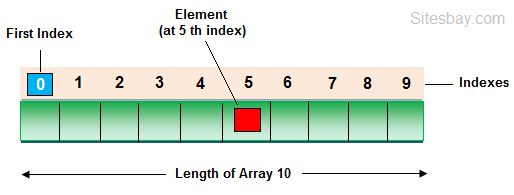# Array in PHP

## Array in PHP

In PHP Array is used to store multiple values in single variable. An array is a special variable, which can hold more than one value at a time.### Create an Array in PHP

In PHP, the array() function is used to create an array.

## Array Syntax

```array();
```

### Types of Array in PHP

There are three types of array in php, which are given below.

• Indexed arrays - Arrays with a numeric index
• Associative arrays - Arrays with named keys
• Multidimensional arrays - Arrays containing one or more arrays

### Indexed Arrays

The index can be assigned automatically (index always starts at 0), you can see in below example;

## Array Example

```<?php

\$student = array("Harry", "Varsha", "Gaurav");
echo "Class 10th Students " . \$student . ", " . \$student . " and " . \$student . ".";

?>
```

## Output

```Class 10th Students Harry, Varsha and Gaurav
```

### Find Length of an Array in PHP

Using count() function you can find length of an array in php.

## Array Example

```<?php

\$student = array("Harry", "Varsha", "Gaurav");
echo "Length of Array: ";
echo count(\$cars);

?>
```

## Output

```Length of Array: 3
```

## Array Example

```<?php

\$student = array("Harry", "Varsha", "Gaurav");
\$arrlength = count(\$student);

for(\$i = 0; \$i < \$arrlength; \$i++)
{
echo \$student[\$i];
echo "<br>";
}

?>
```

## Output

```Harry
Varsha
Gaurav
```

### Associative Arrays in PHP

In this type of array; arrays use named keys that you assign to them.

## Syntax

```\$age = array("Harry"=>"10", "Varsha"=>"20", "Gaurav"=>"30");

or

\$age['Harry'] = "10";
\$age['Varsha'] = "20";
\$age['Gaurav'] = "30";
```

### Multidimensional Arrays in PHP

A multidimensional array is an array containing one or more arrays. For a two-dimensional array you need two indices to select an element

## Array Example

```<?php
\$student = array
(
array("Harry",300,11),
array("Varsha",400,10),
array("Gaurav",200,8),
array("Hitesh",220,8)
);

\$student = array("Harry", "Varsha", "Gaurav");
echo \$student.": Marks: ".\$student.", Class: ".\$student.".<br>";
echo \$student.": Marks: ".\$student.", Class: ".\$student.".<br>";
echo \$student.": Marks: ".\$student.", Class: ".\$student.".<br>";
echo \$student.": Marks: ".\$student.", Class: ".\$student.".<br>";

?>
```

## Output

```Harry: Marks: 300 Class: 11
Varsha: Marks: 400 Class: 10
Gaurav: Marks: 200 Class: 8
Hitesh: Marks: 220 Class: 8
```